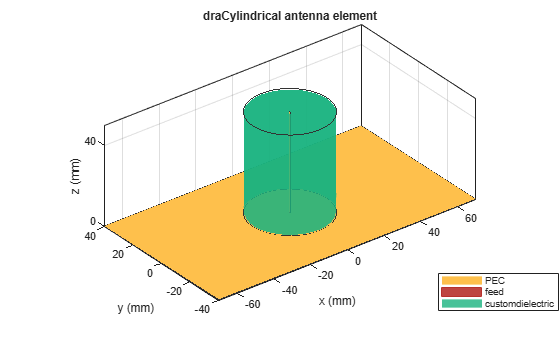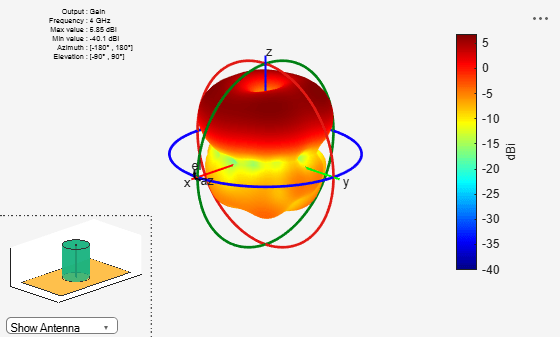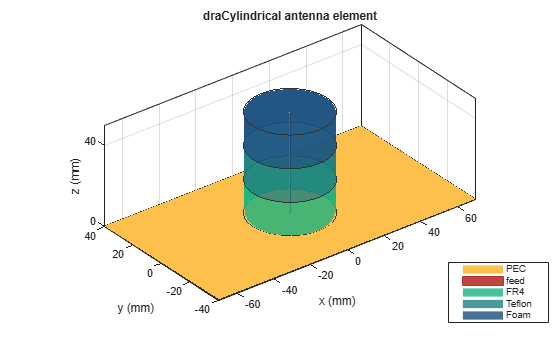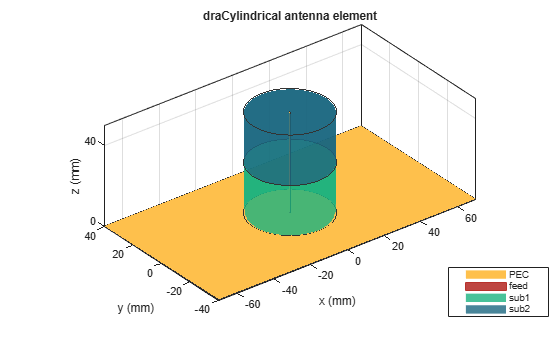# draCylindrical

Create cylindrical dielectric resonator antenna

## Description

The `draCylindrical` object creates a cylindrical dielectric resonator antenna. The cylindrical dielectric resonator antenna consists of a cylindrical dielectric placed on the ground plane. It has high power-handling capacity and can provide high gain and bandwidth. These antennas are more suitable for use at microwave frequencies. Cylindrical dielectric resonator antennas are widely used in medium- and long-range communications.## Creation

### Syntax

``ant = draCylindrical``
``ant = draCylindrical(Name,Value)``

### Description

example

````ant = draCylindrical` creates a cylindrical dielectric resonator antenna with dimensions for a resonant frequency of 1.5 GHz. The default antenna is probe fed with the feedpoint at the origin.```

example

````ant = draCylindrical(Name,Value)` sets Properties using one or more name-value pairs. For example, `draCylindrical('ResonatorRadius',0.04)` creates a cylindrical dielectric resonator antenna with the radius of the dielectric resonator set to 40 mm.```

## Properties

expand all

Radius of the dielectric resonator, specified as a positive scalar in meters.

Example: `'ResonatorRadius',0.05`

Data Types: `double`

Type of dielectric material used as a substrate, specified as a dielectric material object. You can choose any material from the `DielectricCatalog` or use your own dielectric material. For more information, see `dielectric`. For more information on dielectric substrate meshing, see Meshing.

Note

The substrate dimensions must be lesser than the ground plane dimensions.

Example: `d = dielectric('FR4'); 'Substrate',d`

Example: ```d = dielectric; d.Name = 'sub1'; d.EpsilonR = 2.3; d.LossTangent = 0.002; d.Thickness = 0.01; ant.Substrate = d;```

Ground plane length, specified as a positive scalar in meters. By default, ground plane length is measured along the x-axis. Set `'GroundPlaneLength'` to `Inf` to use the infinite ground plane technique for antenna analysis.

Example: `'GroundPlaneLength',120e-3`

Data Types: `double`

Ground plane width, specified as a positive scalar in meters. By default, ground plane width is measured along the y-axis. Set `'GroundPlaneWidth'` to `Inf` to use the infinite ground plane technique for antenna analysis.

Example: `'GroundPlaneWidth',118e-3`

Data Types: `double`

Width of the feed, specified as a positive scalar in meters.

Example: `'FeedWidth',5e-05`

Data Types: `double`

Height of the feed, specified as a positive scalar in meters.

Example: `'FeedHeight',0.060`

Data Types: `double`

Signed distance of the feedpoint from the center of the ground plane, specified as a two-element vector in meters.

Example: `'FeedOffset',[–0.0070 0.01]`

Data Types: `double`

Type of the metal used as a conductor, specified as a metal material object. You can choose any metal from the `MetalCatalog` or specify a metal of your choice. For more information, see `metal`. For more information on metal conductor meshing, see Meshing.

Example: `m = metal('Copper'); 'Conductor',m`

Example: `m = metal('Copper'); ant.Conductor = m`

Tilt angle of the antenna, specified as a scalar or vector with each element unit in degrees. For more information, see Rotate Antennas and Arrays.

Example: `'Tilt',90`

Example: `ant.Tilt = 90`

Example: `'Tilt',[90 90]`,```'TiltAxis',[0 1 0;0 1 1]``` tilts the antenna at 90 degrees about the two axes defined by the vectors.

Data Types: `double`

Tilt axis of the antenna, specified as:

• Three-element vector of Cartesian coordinates in meters. In this case, each coordinate in the vector starts at the origin and lies along the specified points on the X-, Y-, and Z-axes.

• Two points in space, each specified as three-element vectors of Cartesian coordinates. In this case, the antenna rotates around the line joining the two points in space.

• A string input describing simple rotations around one of the principal axes, 'X', 'Y', or 'Z'.

For more information, see Rotate Antennas and Arrays.

Example: `'TiltAxis',[0 1 0]`

Example: `'TiltAxis',[0 0 0;0 1 0]`

Example: `ant.TiltAxis = 'Z'`

Lumped elements added to the antenna feed, specified as a `lumpedElement` object handle. You can add a load anywhere on the surface of the antenna. By default, the load is at the feed. For more information, see `lumpedElement`.

Example: `'Load',lumpedelement`, where `lumpedelement` is the load added to the antenna feed.

Example: `ant.Load = lumpedElement('Impedance',75)`

## Object Functions

 `show` Display antenna, array structures or shapes `axialRatio` Axial ratio of antenna `beamwidth` Beamwidth of antenna `charge` Charge distribution on antenna or array surface `current` Current distribution on antenna or array surface `design` Design prototype antenna or arrays for resonance around specified frequency `efficiency` Radiation efficiency of antenna `EHfields` Electric and magnetic fields of antennas; Embedded electric and magnetic fields of antenna element in arrays `impedance` Input impedance of antenna; scan impedance of array `mesh` Mesh properties of metal, dielectric antenna, or array structure `meshconfig` Change mesh mode of antenna structure `optimize` Optimize antenna or array using SADEA optimizer `pattern` Radiation pattern and phase of antenna or array; Embedded pattern of antenna element in array `patternAzimuth` Azimuth pattern of antenna or array `patternElevation` Elevation pattern of antenna or array `rcs` Calculate and plot radar cross section (RCS) of platform, antenna, or array `returnLoss` Return loss of antenna; scan return loss of array `sparameters` Calculate S-parameter for antenna and antenna array objects `vswr` Voltage standing wave ratio of antenna

## Examples

collapse all

Create a cylindrical dielectric resonator antenna with default properties.

`ant = draCylindrical`
```ant = draCylindrical with properties: ResonatorRadius: 0.0200 Substrate: [1x1 dielectric] GroundPlaneLength: 0.1400 GroundPlaneWidth: 0.0800 FeedWidth: 1.0000e-03 FeedHeight: 0.0500 FeedOffset: [0 0] Conductor: [1x1 metal] Tilt: 0 TiltAxis: [1 0 0] Load: [1x1 lumpedElement] ```

View the antenna using the `show` function.

`show(ant)`Plot the radiation pattern of the cylindrical dielectric resonator antenna at a frequency of 4 GHz.

`pattern(ant,4e9)`Create a cylindrical dielectric resonator antenna with FR4, Teflon, and foam as substrates.

```ant = draCylindrical; d = dielectric('FR4','Teflon','Foam'); d.Thickness = [ant.Substrate.Thickness/3 ant.Substrate.Thickness/3 ant.Substrate.Thickness/3]; ant.Substrate = d; ant = draCylindrical('Substrate',d); show(ant)```Create a cylindrical dielectric resonator antenna with substrates having relative permittivity as 2.3 and 4.5, respectively. The value of loss tangent for both the substrates is 0.002.

```ant = draCylindrical; d = dielectric; d.Name = {'sub1','sub2'}; d.EpsilonR = [2.3 4.5]; d.LossTangent = [0.002 0.002]; d.Thickness = [ant.Substrate.Thickness/2 ant.Substrate.Thickness/2]; ant.Substrate = d; show(ant)```